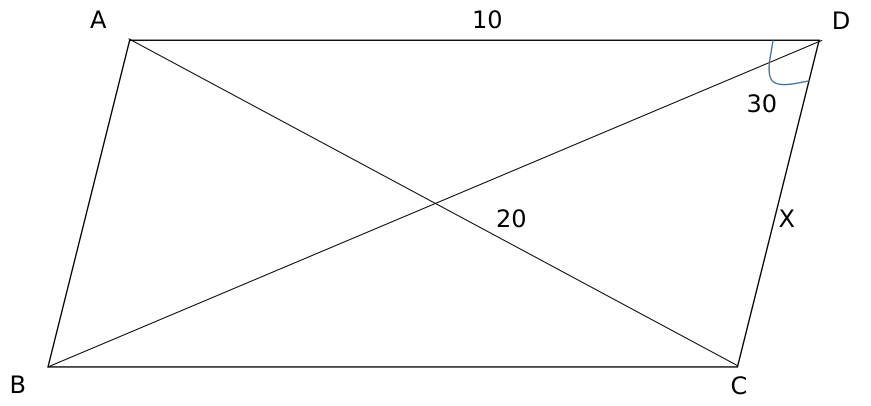Question 65

# Let ABCD be a parallelogram. The lengths of the side AD and the diogonal AC are 10cm and 20cm, respectively. If the angle $$\angle ADC$$ is equal to $$30^{0}$$ then the area of the parallelogram, in sq.cm is

SolutionApplying cosine rule in triangle ACD,

$$100+X^2-2\times\ 10\times\ X\cos30=400$$

$$X^2-10X\sqrt{\ 3}-300=0$$

Solving, we get X = $$\left(\frac{10\sqrt{\ 3}+10\sqrt{\ 15}}{2}\right)$$

Hence, area = 10Xsin 30 = $$\frac{\left(\frac{10\sqrt{\ 3}+10\sqrt{\ 15}}{2}\right)10}{2}$$

= $$25(\sqrt{3}+\sqrt{15})$$